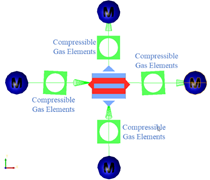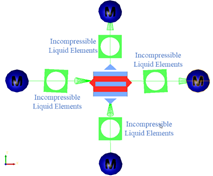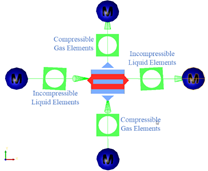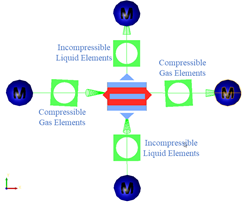# Generic Heat Exchanger Component

## Description

The Generic Heat Exchanger requires that the heat exchanger performance is known. There are several options to specify the performance such as: temperature change, thermal duty, NTU, effectiveness, and the Hs parameter.

Generic Heat Exchanger can be used in Compressible and Incompressible (hydraulic and non-hydraulic) simulations. Currently no phase change is available. The component has four fluid connections, and it models heat exchange between two streams in a network. Heat Exchangers in Flow Simulator come with 4 hidden chambers representing the 4 sides of a heat exchanger. The Generic Heat Exchanger uses Orifice or Effective Area elements (Compressible or Incompressible) in the backend to model restrictions losses (pressure loss) of both flow paths based on user defined loss parameters and characteristic flow area. The Heat addition/removal is calculated based on user inputs for Heat Exchanger Performance curves in Generic Hx module and Q (Heat Addition/Removal) is supplied to Orifice or Effective Area Elements to predict temperatures of the exiting fluids.

Some of the important modeling aspects to be taken care while using heat exchanger components are:
• The Hot Side circuit must get connected to Line 1 (Indicated by Red Color part of Component Image) and Cold Side circuit get connected to Line 2 (Indicated with Blue Color part of Component Image) as shown in the image below.
• You can choose to model additional inlet and outlet losses using the separate Discrete Loss/Tube Element upstream and downstream of heat exchanger respectively.
• The Hot/Cold Side circuit line must be connected with either compressible or Incompressible set of elements. Mixing of elements sets for a Hot/Cold side circuit line is not allowed. Below Table represents some modeling/allowable scenarios.
Modeling/Allowable Scenarios Flow Simulator Network
Hot Side: Compressible Gases

Cold Side: Compressible Gases

Example: Air to Air Heat ExchangerHot Side : Incompressible Liquids

Cold Side : Incompressible Liquids

Example: Fuel Cooled Oil Cooler (FCOC)Hot Side: Incompressible Liquids

Cold Side: Compressible Gases

Example: Air Cooled Oil Cooler (ACOC)Hot Side: Compressible Gases

Cold Side: Incompressible Liquids

Example: Air to Liquid Heat ExchangerFlow Simulator can also be used for Heat Exchanger design. For heat exchanger design a detailed representation of the heat exchanger is required. The example below shows flow elements representing the cold and hot side with thermal network resistors connecting the sides. Heat exchanger performance can be predicted from such a model.

## Generic Heat Exchanger Element Inputs

Table of the inputs for the Generic Heat Exchanger Component.

Element Specific Generic Heat Exchanger Component Input Variables
Index UI Name (.flo label) Description
2,10 Geometric Input Type (CS_SHAPE_COLD, CS_SHAPE_HOT) Type of geometry information:

0. Enter Pipe Area only (assume Hydraulic Diameter from assumed circular shape).

1. Enter Pipe Area and Hydraulic Diameter.

3,11 Cross Sectional Area

(AREA_COLD, AREA_HOT)

Flow area for hot and cold side fluids.
4,12 Hydraulic Diameter

(HYD_DIA_COLD, HYD_DIA_HOT)

Hydraulic Diameter for hot and cold side fluids.
5,13 Pressure Loss Options

(PLOSS_COLD, PLOSS_HOT)

Options to specify type of pressure loss modeling:

1. Fixed Loss Coefficient

2. Fixed Total Pressure Drop

3. Flow vs. Delta.P (PTIN - PSEX)

4. Velocity vs. Delta.P (PTIN - PSEX)

5. Loss Coefficient vs. Reynolds Number

16. Flow vs. Delta P vs. Temperature

6,14 Loss Coefficient

(KLOSS_COLD, KLOSS_HOT)

Incompressible Loss Coefficient
7,15 Delta Total Pressure

(DELTA_PT_COLD, DELTA_PT_HOT)

Total pressure drop across a flow path.
8,16 Mass or Volume (MASS_VOL_COLD, MASS_VOL_HOT) Type of flow input for PLOSS = 3 and 16

0. Flow in lbm/sec

1. Flow in gallons per minute

9,17 Mass or Volume

(NPRCD_COLD, NPRCD_HOT)

Number of values in PLOSS tables
18-24   Not used
25 Heat Transfer Options (HOPT) Options for modeling Heat Transfer between Hot and Cold side fluids:

1. Thermal Duty: Heat Input

2. Hot Fluid Delta.T

3. Cold Fluid Delta.T

4. Effectiveness

5. Effectiveness vs. Flow_Rate_Cold vs. Flow_Rate_Hot

8. Effectiveness vs. NTU vs. Heat Capacity Ratio

9. Nusselt Number vs. RE_Cold vs. RE_Hot

10. Constant hA coefficient value

14. Hs Constant

15. Hs vs Flow_Rate_Cold vs. Flow_Rate_Hot

26 Heat Input (QIN) Heat Addition
27 Fluid Delta.T (DELT_T) Delta Total Temperature for Hot (or Cold) Side fluid
28 Effectiveness (EFFECTIVENESS) Effectiveness of Heat Exchanger
29 Type of Configurations

(CONFIG_TYPE)

Heat Exchangers Flow Configurations

0. Parallel Flow

1. Counter Flow

2. Shell and Tube

3. Cross flow both flows unmixed

Cross flow One fluid mixed

30 Number of Shell Passes (NUM_SHELL_PASS) No. of Shell Passes for Shell and Tube Hx Configurations
31 Primary hA coefficient (PRI_HA) Htc*Area Coefficient of Primary side fluid
32 Secondary hA coefficient (SEC_HA) Htc*Area Coefficient of Secondary side fluid
33,34   Not used
35 Mass or Volume

(MASS_OR_VOL)

Type of flow input for HOPT = 5 and 15

0. Flow in lbm/sec

1. Flow in gallon per minute

36 Overall HTC

(HTC_OPT)

Type of HTC input for HOPT=8

1. Fixed Overall HTC

2. Nusselt Number vs. Re Cold and Hot

37 Overall Heat Transfer Coefficient (OVERALL_HTC) Overall Heat Transfer Coefficient Input
38,39,43,44,45 (NHT, NHT_1, NHT_2, NHT_3, NHT_4) Table sizes
40 Select Mixed Flow (MIXED_FLOW) 4. Mixed Flow side of HX for Cross flow one side mixed option.

1=Primary, 2=Secondary

41 HX Area (HX_AREA) The total heat exchanger area to be used with the Hs performance parameter
42 Hs Parameter

(HS_PARAMETER)

A Heat Exchanger Performance Parameter
T1, T2, T3, T4 Flow vs Delta P

(COLD_DELTA-P, COLD_FLOW,

HOT_DELTA-P, HOT_FLOW)

• User-defined curve for Flow vs. Delta.P. Delta-P table is the difference between the upstream driving total pressure and downstream sink static pressure.
T1, T2, T3, T4 Fluid Velocity vs Delta P (COLD_DELTA-P, COLD_VEL,

HOT_DELTA-P, HOT_VEL)

User-defined curve for Fluid Velocity vs. Delta.P. Delta-P table is the difference between the upstream driving total pressure and downstream sink static pressure.
T1, T2, T3, T4 Loss Coefficient vs Reynolds Number

(COLD_KLOSS, COLD_REYN,

HOT_ KLOSS, HOT_REYN)

User-defined curve for Loss Coefficient vs. Reynolds Number.

Where:

If Hydraulic Diameter is provided, then:
If Hydraulic Diameter or Perimeter is not Provided, Reynolds number is calculated based on Orifice Area:
T5, T6, T7 Effectiveness vs. Flow_Rate_Cold vs. Flow_Rate_Hot

(FLOWRATE_COLD, FLOW_HOT.., EFFECTIVENESS)

3D Table for Effectiveness vs. Flow_Rate_Cold vs Flow_Rate_Hot
T5, T6, T7 Effectiveness vs. NTU vs Heat Capacity Ratio

(NTU, ..HEAT_RATIO, EFFECTIVENESS)

3D Table for Effectiveness vs. NTU vs Heat Capacity Ratio
T8, T9, T10 Nusselt Number vs. RE_Cold vs. RE_Hot

(NusseltNumber, ReynoldsCold, ReynoldsHot)

3D Nusselt Number vs. RE_Cold vs RE_Hot
T1, T2, T11 Cold Flow vs. Delta P vs. T

(COLD_DELTA-P, COLD_FLOW, COLD_FLOW_T)

3D Table for Cold Flow vs. Delta P vs. T
T3, T4, T12 Hot Flow vs. Delta P vs. T

(HOT_DELTA-P, HOT_FLOW, HOT_FLOW_T)

3D Table for Hot Flow vs. Delta P vs. T

## Generic Heat Exchanger Theory Manual

Nomenclature:
$W$ : Mass flow rate C: Heat Capacity
$\rho$ : Density Q: Heat Addition/Rejection
$Cp$ : Specific Heat Tt: Total Temperature
NTU: Number of Transfer Units
Subscripts:
in, up, 1: Upstream station C: Cold
ex, dn, 2: Downstream station H: Hot

Pressure Loss Calculations

There are six different options through which pressure loss across a Hot or Cold stream can be modeled. They are:

1. Fixed Loss Coefficient
2. Fixed Total Pressure Drop
3. Flow vs Delta.P (PTIN – PSEX)
4. Velocity vs Delta.P (PTIN – PSEX)
5. Loss Coefficient vs Reynolds Number
6. Flow vs Delta P vs Delta T

As discussed above, Generic Heat Exchanger uses Orifice or Effective Area elements (Compressible or Incompressible) in the backend to model restrictions losses (pressure loss).

To get more details on pressure drop calculation for:
• Fixed Loss Coefficient and Fixed Pressure Drop input refer to the Orifice Documentation.
• Curve based inputs (3-6) mentioned above refer to the Effective-Area Orifice Documentation

Heat Transfer Calculations

1. Heat Input (Qin)

${T}_{t,ex,Cold}={T}_{t,in,Cold}+\frac{Qin}{{W}_{Cold}*C{p}_{avg,Cold}}$

${T}_{t,ex,Hot}={T}_{t,in,Hot}-\frac{Qin}{{W}_{Hot}*C{p}_{avg,Hot}}$

2. Hot Fluid Delta.T

${T}_{t,ex,Cold}={T}_{t,in,Cold}+\frac{Q}{{W}_{Cold}*C{p}_{avg,Cold}}$

3. Cold Fluid Delta.T

${T}_{t,ex,Hot}={T}_{t,in,Hot}-\frac{Q}{{W}_{Hot}*C{p}_{avg,Hot}}$

4. Effectiveness

${T}_{t,ex,Cold}={T}_{t,in,Cold}+\frac{Q}{{W}_{Cold}*C{p}_{avg,Cold}}$

${T}_{t,ex,Hot}={T}_{t,in,Hot}-\frac{Q}{{W}_{Hot}*C{p}_{avg,Hot}}$

5. Effectiveness vs Flow_Rate_Cold vs Flow_Rate_Hot

Effectiveness is obtained from User Defined Input for Effectiveness as function Flow_Rate_Cold and Flow_Rate_Hot.

${T}_{t,ex,Cold}={T}_{t,in,Cold}+\frac{Q}{{W}_{Cold}*C{p}_{avg,Cold}}$

${T}_{t,ex,Hot}={T}_{t,in,Hot}-\frac{Q}{{W}_{Hot}*C{p}_{avg,Hot}}$

6. Effectiveness vs NTU vs Heat Capacity Ratio

Effectiveness is obtained from User Defined Input for Effectiveness as function NTU and Heat Capacity Ratio.

UA is calculated from Constant user input or from curve specified for Nusselt Number as function of Reynolds Number Cold and Reynolds Number Hot

$Effectiveness=1-{e}^{\left(\left(1}{{C}_{Ratio}}\right)NT{U}^{0.22}{e}^{\left(-{C}_{Ratio}NT{U}^{0.78}-1\right)}\right)}$

${T}_{t,ex,Cold}={T}_{t,in,Cold}+\frac{Q}{{W}_{Cold}*C{p}_{avg,Cold}}$

${T}_{t,ex,Hot}={T}_{t,in,Hot}-\frac{Q}{{W}_{Hot}*C{p}_{avg,Hot}}$

7. Nusselt Number vs RE_Cold vs RE_Hot

Nusselt Number is obtained from User Defined Input for Nusselt Number as function of Reynolds Number Cold and Reynolds Number Hot

$Effectiveness=1-{e}^{\left(\left(1}{{C}_{Ratio}}\right)NT{U}^{0.22}{e}^{\left(-{C}_{Ratio}NT{U}^{0.78}-1\right)}\right)}$

${T}_{t,ex,Cold}={T}_{t,in,Cold}+\frac{Q}{{W}_{Cold}*C{p}_{avg,Cold}}$

${T}_{t,ex,Hot}={T}_{t,in,Hot}-\frac{Q}{{W}_{Hot}*C{p}_{avg,Hot}}$

8. Constant hA Coefficient value

$\frac{1}{UA}={\left(\frac{1}{h*A}\right)}_{Cold}+{\left(\frac{1}{h*A}\right)}_{Hot}$

NTU Effectiveness Methods:
1. Cross Flow Unmixed

$Effectiveness=1-{e}^{\left(\left(1}{{C}_{Ratio}}\right)NT{U}^{0.22}{e}^{\left(-{C}_{Ratio}NT{U}^{0.78}-1\right)}\right)}$

2. Counter Flow

3. Parallel Flow

4. Cross Flow Both Side Mixed

5. Cross Flow One Side Mixed

Cmin is mixed: $Effectiveness=1-{e}^{-\left(1}{{C}_{Ratio}}\right)\left(1-{e}^{-{C}_{Ratio}NTU}\right)}$

Cmax is mixed:

${T}_{t,ex,Cold}={T}_{t,in,Cold}+\frac{Q}{{W}_{Cold}*C{p}_{avg,Cold}}$

${T}_{t,ex,Hot}={T}_{t,in,Hot}-\frac{Q}{{W}_{Hot}*C{p}_{avg,Hot}}$

9. Hs Parameter Methods (Constant and vs Hot and Cold Flowrates)

The Hs parameter is typically used to describe radiators where the hot side is a liquid coolant, and the cold side is air.

${H}_{s}=\frac{ABS\left({W}_{Hot}\right)*C{p}_{avg,Hot}*\left({T}_{h,in}-{T}_{h,ex}\right)}{H{X}_{Area}*\left({T}_{h,in}-{T}_{c,in}\right)}$

${H}_{s}*H{X}_{Area}*\left({T}_{h,in}-{T}_{c,in}\right)=ABS\left({W}_{Hot}\right)*C{p}_{avg,Hot}*\left({T}_{h,in}-{T}_{h,ex}\right)=Q$

A heat exchanger effectiveness can be calculated using the Hs parameter.

$Effectiveness=\frac{{H}_{s}*H{X}_{Area}*\left({T}_{h,in}-{T}_{c,in}\right)}{{C}_{min}*\left({T}_{h,in}-{T}_{c,in}\right)}=\frac{{H}_{s}*H{X}_{Area}}{{C}_{min}}$

Q is found using the effectiveness Qmax. The Q is applied to the fluid streams to get the exit temperatures.

${T}_{t,ex,Cold}={T}_{t,in,Cold}+\frac{Q}{{W}_{Cold}*C{p}_{avg,Cold}}$

${T}_{t,ex,Hot}={T}_{t,in,Hot}-\frac{Q}{{W}_{Hot}*C{p}_{avg,Hot}}$

Note: Bi-Linear interpolation is employed between the values in the table to determine Y Values. If X-Value is less than its first value entry in the table, the first Y-Value entry is used. If X-Value is greater than its last value entry in the table, the last Y-Value entry is used. Flow Simulator doesn’t do any extrapolation if the values are outside the prescribed input limits.

## Generic Heat Exchanger Outputs

The following listing provides details about Generic Heat Exchanger Component output variables.

Name Description Units
PS Static pressure psia, MPa
PT Total pressure psia, MPa
TT Total temperature of fluid deg F, deg K
RE Reynold Number (None)
Rho Density lbm/ft^3, Kg/m^3
CP Specific Heat Btu/(Lbm R), kJ/Kg.K
K Thermal Conductivity Btu/(hr ft R), W/m.K
DVISC Dynamic Viscosity Lbm/(hr ft), N s/m^2
Area Flow Area In2, m2
Heat Mode Heat Mode Options (An echo of the user input)
1. Heat Input
2. Hot Fluid Delta.T
3. Cold Fluid Delta.T
4. Effectiveness
5. Effectiveness vs Flow_Rate_Cold vs Flow_Rate_Hot
6. Effectiveness vs NTU vs Heat Capacity Ratio
7. Nusselt Number vs RE_Cold vs RE_Hot
8. Constant hA coefficient value
(None)
Pri/cold_side_ploss_opt Options specified for Pressure Loss Modeling for Pri/Cold side
1. Fixed Loss Coefficient
2. Fixed Total Pressure Drop
3. Flow vs Delta.P (PTIN – PSEX)
4. Velocity vs Delta.P (PTIN – PSEX)
5. Loss Coefficient vs Reynolds Number

(An echo of the user input)

(None)
Sec/hot_side_ploss_opt Options specified for Pressure Loss Modeling for Sec/Hot side
1. Fixed Loss Coefficient
2. Fixed Total Pressure Drop
3. Flow vs Delta.P (PTIN – PSEX)
4. Velocity vs Delta.P (PTIN – PSEX)
5. Loss Coefficient vs Reynolds Number

(An echo of the user input)

(None)
Q Heat Transferred between Heat Exchanger fluids BTU/sec, W
Effectiveness Effectiveness of Heat Exchanger (None)
Pri/cold_side_delta.p Delta.PT on Pri/Cold side flow psia, MPa
Sec/hot_side_delta.p Delta.PT on Sec/Hot side flow psia, MPa
Mdot Mass Flow Rate Lbm/s, kg/s
Flow configuration Heat Exchangers Flow Configurations
1. Parallel Flow
2. Counter Flow
3. Shell and Tube
4. Cross flow both flows unmixed
5. Cross flow One fluid mixed

(An echo of the user input)

(None)
Overall conductance(UA) Overall Thermal Resistance BTU/hr.F, W/K
NTU No of Transfer Units (None)
Pri_hA_out Pri/Cold side flow hA Coefficient value BTU/hr.F, W/K
Sec_hA_out Sec/Hot side flow hA Coefficient value BTU/hr.F, W/K